JAIC 1980, Volume 19, Number 2, Article 2 (pp. 69 to 74)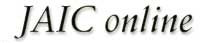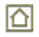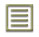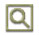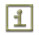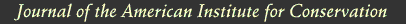JAIC 1980, Volume 19, Number 2, Article 2 (pp. 69 to 74)

### 5 EXPERIMENTAL

Materials: The rag and newsprint papers were commercial papers with cold extraction pH values of 6.7 and 5.0 respectively. The solvents were Fisher, Inc. reagent grade.

Solvent Treatment: Oven-dried samples of paper (45 to 55 mg) were conditioned for 72 hours at 50% RH, 20�C, and placed in beakers containing 25 ml of solvent. After 15 minutes, the samples were removed from the beakers, blotted to remove excess solvent, and dried under the conditions described in Tables I and II.

Solvent Analysis: Although a gravimetric method of analysis is often used to determine the solvent content of cellulosic materials,3–11 such an analysis is applicable only under anhydrous conditions. At 50% RH, both solvent and moisture can contribute to the weight of a paper sample. Thus, we chose an analytical procedure, gas chromatography, capable of distinguishing between moisture and organic solvents.

The paper sample to be analyzed was place in a glass tube fitted with a septum cap, and 150 μl of an extraction solvent, containing 1.00% by volume of an internal standard, was added. The tube was allowed to condition for 48 hours at room temperature to insure complete extraction of the solvent from the paper.6 Following this extraction period, a sample of the solution (about 100 μl) was withdrawn from the tube and injected onto a 15 foot Carbowax 20M column. Table IV shows details of the analysis for each solvent.

TABLE IV ANALYTICAL SYSTEMS FOR SOLVENT DETERMINATION WITH A 15 FOOT CARBOWAX 20M GC COLUMN

Peak heights for the solvent and the internal standard were measured from the GC trace, and the percent solvent content of the original paper sample was calculated as follows.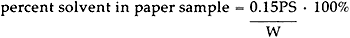In this expression, 0.15 is the volume, in milliliters, of extraction solvent added to the sample tube; P is the height of the solvent peak divided by the height of the internal standard peak; S is the slope of a calibration curve such as that shown in Figure 1; and W is the weight, in grams, of the paper sample.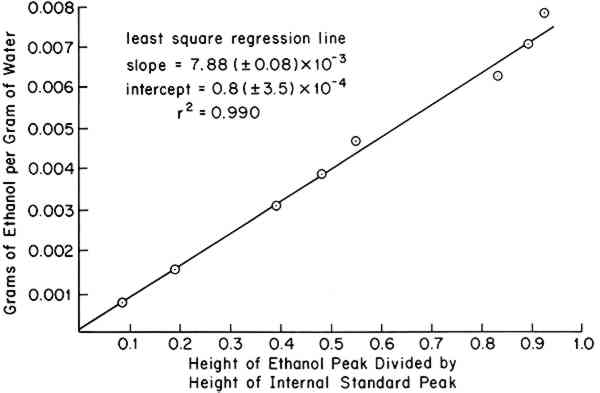Sensitivity and Precision: Figure 1 shows a typical calibration curve obtained from 100 μl injections of standard solutions. The least-squares intercept was found to be 0.8 (�3.5) � 10−4 grams of ethanol per gram of water (95% confidence interval). Assuming a 50 mg paper sample is analyzed and no peak is observed for ethanol in the GC trace, the maximum value of PS in the above equation would be 4.3 � 10−4 (maximum intercept). From the equation, this represents 0.13% ethanol by weight. Thus, the zero solvent contents reported in Table II are uncertain to � 0.13%, or less.

### ACKNOWLEDGEMENTS

THIS RESEARCH was supported by a grant from the Andrew W. Mellon Foundation.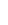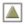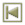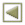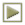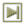Copyright � 1980 American Institute of Historic and Artistic Works